# 范围内汇总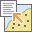范围内汇总工具可计算输入图层与边界图层重叠区域的统计数据。

## 工作流示意图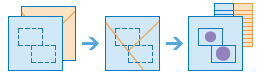## 示例

• 某城市面临维护工程的积压问题，并希望将维护评估地区融入与街灯和自行车车道维护相关的项目中。 范围内汇总工具用于计算每个地区的街灯数和自行车道的英里数，以便有效安排工程的优先级。

• 一家有线电视提供商即将启动一项试点工程，该工程可使收入较低的社区大学的学生以较低的成本访问互联网。 范围内汇总工具用于确定各学区内低收入家庭的数量，以便有线电视提供商可以选择合适的地区来实施其试点工程。

• 一家开发公司正在考虑在城区中心打造综合性开发项目。 该公司可以使用范围内汇总来汇总城市边界内具有资格的区域，以获得潜在开发区的总范围。

## 用法说明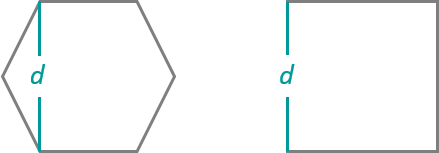• 英里
• 英尺
• 公里

• 平方英里
• 平方千米
• 平方米
• 公顷
• 英亩

## 局限性

• 边界输入必须是面要素。
• 线和面是按比例进行汇总的；汇总线或面时，最好汇总绝对数据（如人口）而非相对数据（如平均收入）。
• 无法选择移除与任何点、线或面都不重叠的边界。

## 范围内汇总工作原理

### 等式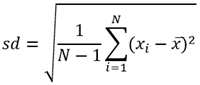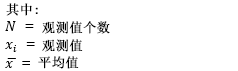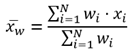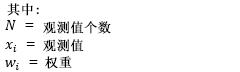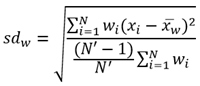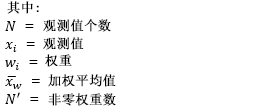### 点

``280 + 408 + 356 + 361 + 450 + 713 = 2,568``

``[280, 408, 356, 361, 450, 713] = 280``

``[280, 408, 356, 361, 450, 713] = 713``

``2,568/6 = 428``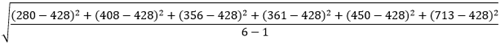``= 150.79``

### 线

``400 + 500 = 900``

``[400, 500] = 400``

``[400, 500] = 500``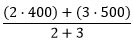``= 460``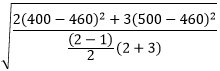``= 69.28``

### 面

``2,133 + 3,133 + 400 + 3,375 + 1,800 = 10,841``

``[2,133, 3,133, 400, 3,375, 1,800] = 400``

``[2,133, 3,133, 400, 3,375, 1,800] = 3,375``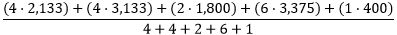``= 2,665.53``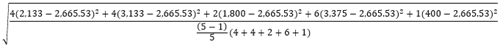``= 925.91``